# New bridge

Thanks to the new bridge, the road between A and B has been cut to one third and is now 10km long. How much did the road between A and B measure before?

x =  30 km

### Step-by-step explanation:

x/3 = 10

x = 30

Our simple equation calculator calculates it.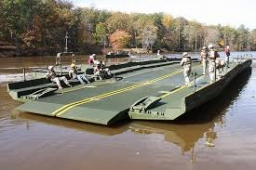Did you find an error or inaccuracy? Feel free to write us. Thank you!Tips to related online calculators
Need help to calculate sum, simplify or multiply fractions? Try our fraction calculator.
Do you have a linear equation or system of equations and looking for its solution? Or do you have a quadratic equation?

## Related math problems and questions:

• Clock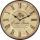What time is now if the time elapsed afternoon is 2/10 of time that elapses before midnight?
• The tourist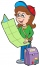The tourist has walked 3/4 of the route. The destination is 12.5 km away. How many kilometers did the route measure?
• QuizQ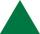An isosceles triangle has two sides of length 7 km and 39 km. How long is a third side?
• Charles Bridge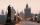Charles Bridge was 15 times older than Frantův bridge from 1900 in 1931. How old is the Charles Bridge?
• Car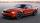The car goes from point A to point B at speed 86 km/h and back 53 km/h. If it goes there and back at speed 67 km/h trip would take 10 minutes shorter. What is the distance between points A and B?
• Leaking swimming pool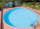Between 10 PM and 7:10 AM, the water level in a swimming pool decreased by 5/12 inches . Assuming that the water level decreased at a constant rate, how much did the water level drop each hour?
• Cyclo tripEighth-graders took a full-day trip on a cycling course. They took 1/7 of the route to the first break and added another 3/7 of the route to lunch. They had 18 km left to the finish. How many kilometers did the trip route measure?
• Bridge piers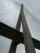One quarter of the bridge pier is sunk into the ground. Two thirds are in the water. Protruding above the water is 1.20 m long. Determine the height of bridge piers.
• Three paintersThe three painters have painted bridge. The first would work done in 5 days, the second in 6 days, and the third in 7.5 days. How long will the bridge work if they work together?
• Motion problemFrom Levíc to Košíc go car at speed 81 km/h. From Košíc to Levíc go another car at speed 69 km/h. How many minutes before the meeting will be cars 27 km away?
• The tourist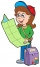The tourist walked a quarter of the way on the first day, a third of the rest on the second day, and 20 km on the last day. How many km did he walk in three days?
• Cars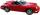Cars started at 7:00 AM. From point A car run at 40 km per hour, from B car run 70 km per hour. Between A and B is a 250 km long road. When and where will meet (measured from point A)?
• Long drive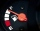Calculate how much it costs to drive 150,000 km with an average consumption of 7 liters per 100 km and a fuel price of 1.2 € / l.
• The ship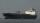The ship went upstream speed of 20 km/h in relation to water. River flows at speed 10 km/h. After half an hour, he stopped and returned downstream to the starting point. How long it took way back when even if downstream speed of ship is 20 km/h in relatio
• DriverI drive at an average speed of 70km/h, I drive 10 thousand km. How long did it take me?
• Cutting pipe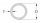A 10⅔ inch long pipe is cut into 8 equal pieces. How long is each piece?
• Pencil cut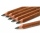Veronika cut 10cm pencil. Each turn, the pencil was reduced by 0.2mm. She turned the pencil 100 times. How many centimeters has a pencil now?